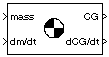# Estimate Center of Gravity

Calculate center of gravity location

• Library:
• Aerospace Blockset / Mass Properties

•## Description

The Estimate Center of Gravity block calculates the center of gravity location and the rate of change of the center of gravity.

Linear interpolation is used to estimate the location of the center of gravity as a function of mass. The rate of change of the center of gravity is a linear function of the rate of change of mass.

## Ports

### Input

expand all

Mass, specified as a scalar.

Data Types: `double`

Rate of change of mass, specified as a scalar or three-element vector.

Data Types: | `double`

### Output

expand all

Center of gravity location, returned as a three-element vector.

Data Types: `double`

Rate of the change of center of gravity location, returned as a three-element vector.

Data Types: `double`

## Parameters

expand all

Gross mass of the vehicle, specified as a double scalar.

#### Programmatic Use

 Block Parameter: `fmass` Type: character vector Values: double scalar Default: `'2'`

Empty mass of the vehicle, specified as double scalar.

#### Programmatic Use

 Block Parameter: `emass` Type: character vector Values: double scalar Default: `'1'`

Center of gravity at the gross mass of the vehicle, specified as a three-element vector.

#### Programmatic Use

 Block Parameter: `fcg` Type: character vector Values: 3-element vector Default: ```[1 1 1]'```

Center of gravity at the empty mass of the vehicle, specified as a three-element vector.

#### Programmatic Use

 Block Parameter: `ecg` Type: character vector Values: 3-element vector Default: ```[0.5 0.5 0.5]'```

## Version History

Introduced before R2006a